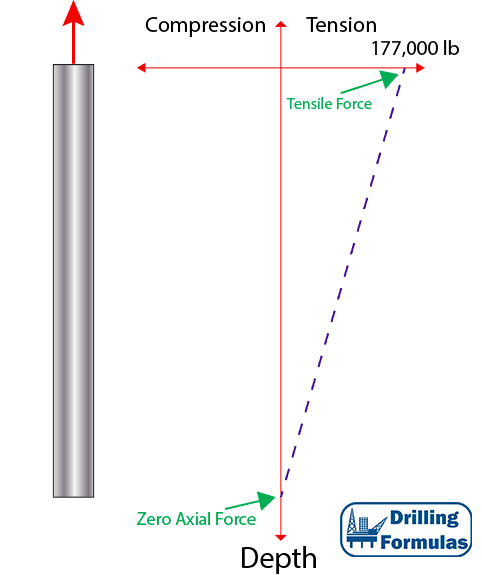# Buoyancy Effect on Weight of Tubular Submersed in Fluid

When a tubular submerses into drilling fluid or completion fluid, it will affect how the force distribution works on the tubular. This article will describe how buoyancy will affect tubular weight and a location of neutral axial load (zero axial load).

Weight of Tubing in Air

When tubing is hung in the air, the string weight is equal to linear weight per foot multiplied by the total length of the string. The maximum tensile load is on surface and the zero axial load point is at the bottom.

Tubing Detail

• 5” Tubing
• Weight per length = 17.7 lb/ft
• Total Length = 10,000 ft

Total weight = 17.7 x 10,000 = 177,000 lb

Weight at the bottom of tubing is 0 and weight on top is 177,000 lb in tensile.

Figure 1 demonstrates force distribution of tubing hanging in air.Figure 1 – Weight of Tubing in Air

Weight of Tubing When Submersed in Fluid

When tubing is submersed in fluid, buoyancy force from fluid, which acts upwards against the cross sectional area of the tubular, generates compression force. This will change the force distribution on the pipe.

Force from buoyancy is based on the equation below;

FB = PH x (Ao-Ai)

PH = 0.052 x M x TVD

Where;

FB = Buoyancy force in lb

PH = Hydrostatic pressure in psi

Ao = Cross sectional area of outer diameter in in2

Ai = Cross sectional area of inner diameter in in2

MW = weight of fluid (mud weight) in pound per gallon (ppg)

TVD = True vertical depth of the well in ft

Figure 2 shows how the buoyancy force acts against the cross sectional area of tubing.Figure 2 – Buoyancy Force Diagram

Tubing Detail

• 5” Tubing
• ID of tubing = 3.696”
• Weight per length = 17.7 lb/ft
• Total Length = 10,000 ft
• Fluid density = 10 ppg

PH = 0.052 x 10 x 10,000 = 5,200 psi

FB = 5,200 x (15.9 – 10.73) = 26,884 lb (compression ⇑(+))

At the bottom of tubing submersed in 10.0 ppg mud, 26,884 lb compression force pushes up against the pipe. This force will reduce tensile force due to the weight of the pipe in the air. The force at the top of tubing is equal to a summation of tensile force caused by tubing weight and compression force acting on the cross sectional area of pipe.

Weight of pipe (lb) = Weight of pipe in air (tensile force) + Compression force due to buoyancy

Weight of pipe (lb) = (-177,000) + (26,884) = -150,116 lb (tensile force ⇓ (-))

Figure 3 demonstrates how force acts against the pipe when it is in fluid. Additionally, it indicates that the zero axial force point is moved up from the bottom to somewhere in the pipe. Below the zero axial force, the pipe is under compression and above this point the pipe is under tension.Figure 3 – Weight of tubing in fluid

Note: for this calculation, the assigned signs and directions of force and pressure are as follows;

Compression force = ⇑(+)

Tensile force = ⇓(-)

Shorten in Length = (-)

Elongate in length = (+)

The signs are based on the Lubinski study.

Lubinski, A., & Althouse, W. S. (1962, June 1). Helical Buckling of Tubing Sealed in Packers. Society of Petroleum Engineers. doi:10.2118/178-PA

References

Jonathan Bellarby, 2009. Well Completion Design, Volume 56 (Developments in Petroleum Science). 1 Edition. Elsevier Science.

Wan Renpu, 2011. Advanced Well Completion Engineering, Third Edition. 3 Edition. Gulf Professional Publishing.

Ted G. Byrom, 2014. Casing and Liners for Drilling and Completion, Second Edition: Design and Application (Gulf Drilling Guides). 2 Edition. Gulf Professional Publishing.

Lubinski, A., & Althouse, W. S. (1962, June 1). Helical Buckling of Tubing Sealed in Packers. Society of Petroleum Engineers. doi:10.2118/178-PA

Share the joy